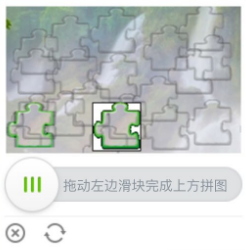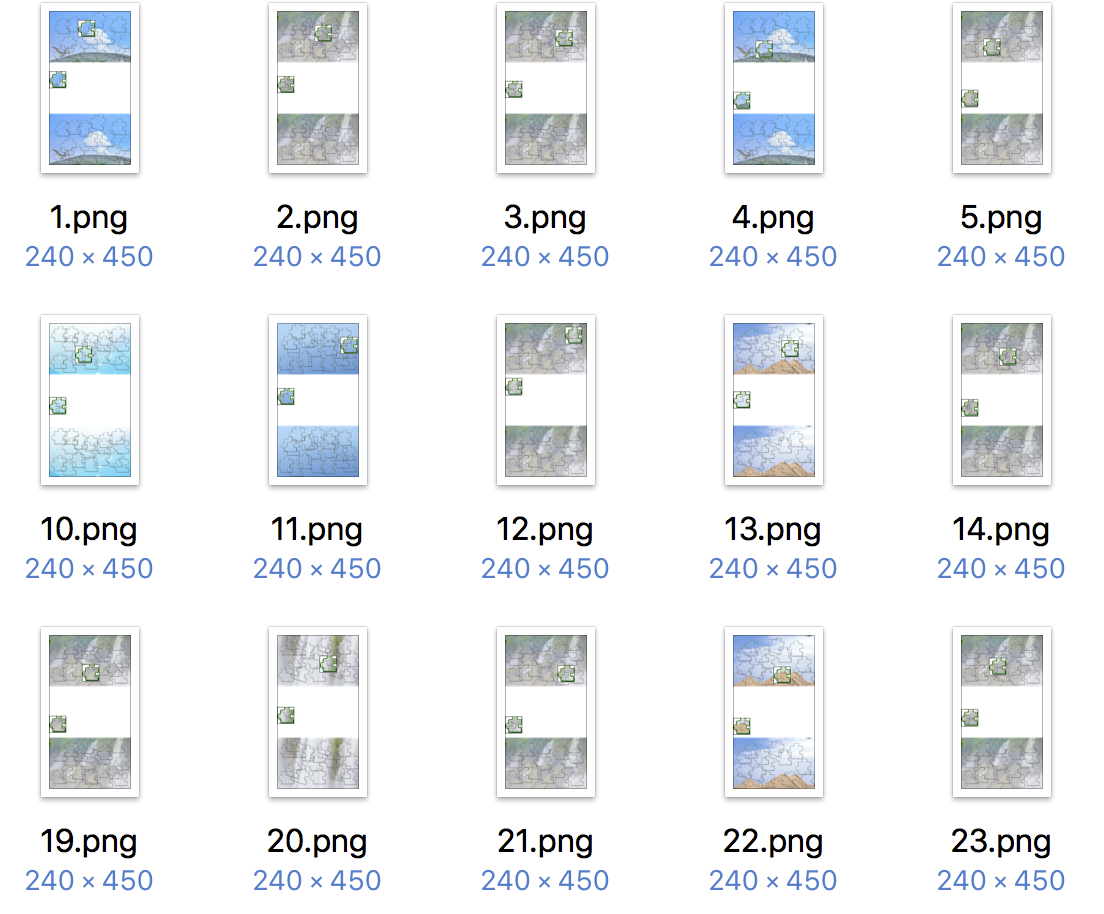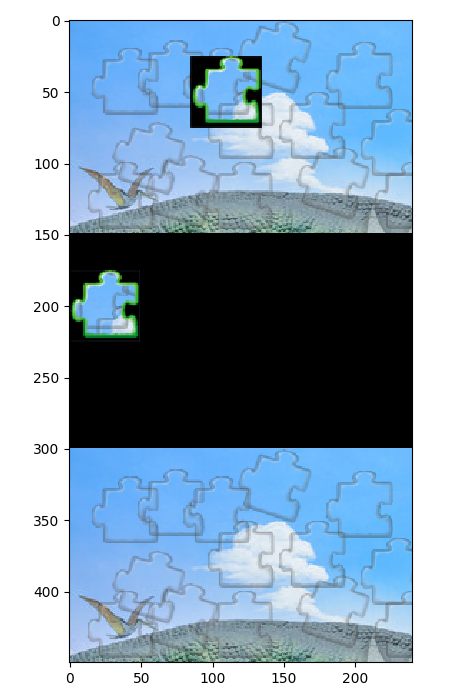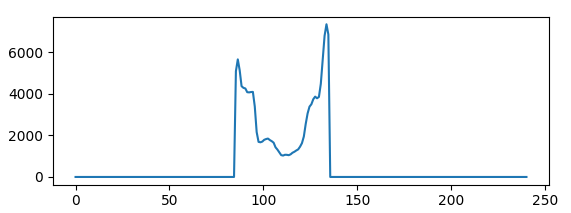# Python实现图片滑动式验证识别方法

1 abstract

2 内容概述

3 研究对象4 定性分析A图完全是由B和C合成

5 定量分析6 求解图片“左上” 减去 “右下” 就得到 “左下”的结果。```import numpy as np
...
"""
对灰度图的某个channel做像素统计
"""
max_value = np.max(sum_along_axis)
bound_value = max_value * ratio
bvalue = (sum_along_axis >= bound_value).astype('int8')
return np.where(bvalue != 0)
def get_predict_ans(img):
"""
根据分类出来的图像，找到相应的图像位置
传入二进制的图片，返回答案
:param img:
:return:
"""
nd_img = np.array(img)
w_pos = get_boundary(nd_img, 0) # 根据分布图找到边界位置
return w_pos```

A+## 目前评论：1   其中：访客  1   博主  0

1.学点编程吧 0

能否转载？谢谢！在学点编程吧，这个公众号上，麻烦了